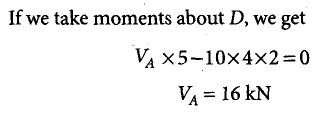Courses

# Test: Structural Analysis & Steel Structures- 1

## 15 Questions MCQ Test GATE Civil Engineering (CE) 2022 Mock Test Series | Test: Structural Analysis & Steel Structures- 1

Description
This mock test of Test: Structural Analysis & Steel Structures- 1 for GATE helps you for every GATE entrance exam. This contains 15 Multiple Choice Questions for GATE Test: Structural Analysis & Steel Structures- 1 (mcq) to study with solutions a complete question bank. The solved questions answers in this Test: Structural Analysis & Steel Structures- 1 quiz give you a good mix of easy questions and tough questions. GATE students definitely take this Test: Structural Analysis & Steel Structures- 1 exercise for a better result in the exam. You can find other Test: Structural Analysis & Steel Structures- 1 extra questions, long questions & short questions for GATE on EduRev as well by searching above.
QUESTION: 1

### A cantilever beam AB, fixed at the end A and carrying a load W at the force end B, is found to deflect by δ at the midpoint of AB. The deflection of B due to load W/2 at the midpoint will be

Solution:

Using Maxwell Reciprocal theorem
Virtual Work Done = Constant

(Load at Mid Point) (Deflection at Mid-Point due to load at B) = (Load at B) (Deflection at B due to load at Mid Point)

(0.5 W) (δ) = ( W) (Deflection at B)
Deflection at B = (δ)/2

QUESTION: 2

### Due to some point load anywhere one fixed beam, the maximum free bending moment is M. The sum of fixed end moments is?

Solution:

Here we will apply the principle of superposition. For a simply supported beam, a concentrated load will form a triangle. The peak of triangle will be M as given in the question,

Area of free bending moment diagram = Area of triangle = 0.5 x M x L

Now, superposing the fixed end moments of supports say M1 and M2. The BMD by these fixed end moments will be a trapezoid with parallel sides having magnitude M1 and M2.

Area of fixed bending moment diagram = Area of trapezoid = - 0.5 x (M1 + M2) x L

As the slopes at the ends of a fixed beam is zero. So, the area of total B.M.D. )fixed end moment + free moment) will be zero.
-0.5 x (M1 + M2) x L = 0.5 x M x L
(M1 + M2) = M

QUESTION: 3

### The bending moment diagram for an overhanging beam is shown in the below figure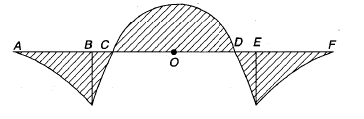The points of contra-flexure for the above beam are

Solution:

Point of contraflexure is the point where bending moment changes its sign. As it can be seen at point C it becomes negative to positive and at point D it becomes positive to negative, therefore, point C and D are points of contraflexure.

QUESTION: 4

A fixed beam of uniform section is carrying a point load at its midspan. When the moment of inertia of the middle half length is reduced to half its original value, then the fixed and moments

Solution:

Fixed end moment due to concentrated load P is given by PL/8.
Since, the fixed end moment does not depend on the cross-sectional properties, hence, it will remain same.

QUESTION: 5

In case of a cantilever beam carrying uniformly varying load, the ratio of the maximum bending moment at free end for conditions, that is, when the load increase from zero at free end to w at fixed end and to that when the load increase from zero at fixed end is

Solution: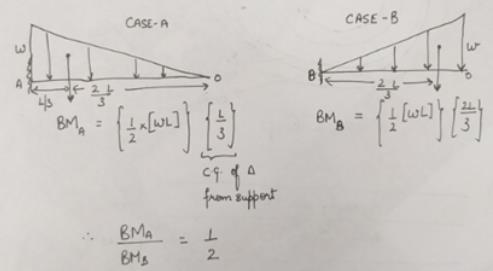QUESTION: 6

A simply supported beam is subjected to an eccentric concentrated load. Where does the maximum deflection of the beam due to the applied load occur?

Solution:

The deflected shape of beam is shown in the figure below. It can be seen that the maximum deflection occurs between the load point and center of the beam.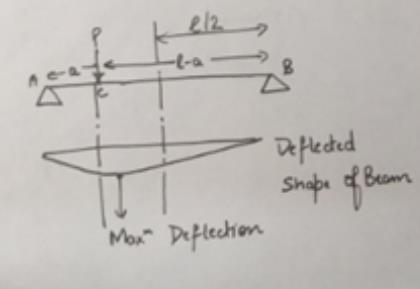QUESTION: 7

A simply supported beam AB of span 1 has a uniform cross section throughout. It carries a central concentrated load W and another load that is uniformly distributed over the entire span, its total magnitude being W. The minimum deflection in the beam is

Solution: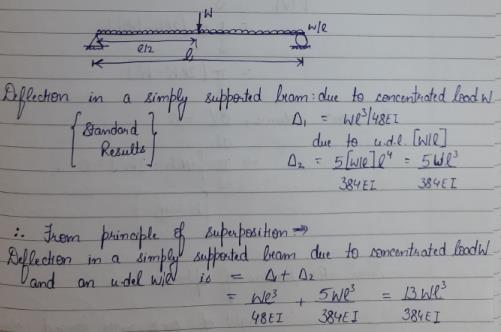QUESTION: 8

For the beam shown below, the collapse load P is given by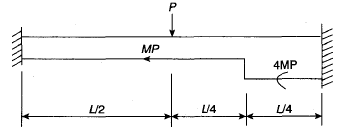Solution: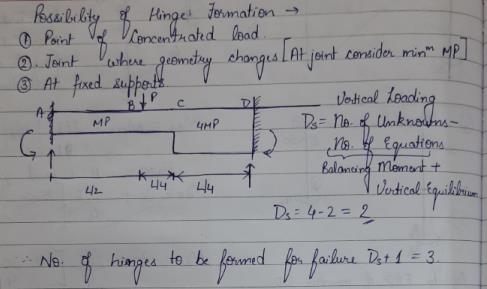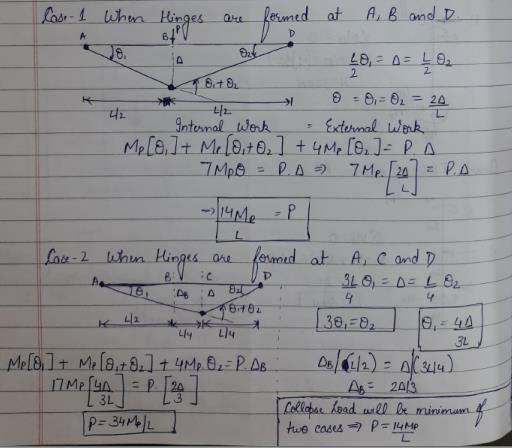QUESTION: 9

The shear force diagram for the portal frame loaded as shown below is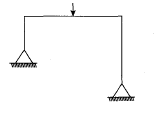Solution:
QUESTION: 10

The influence line diagram for the force in members of the truss shown below is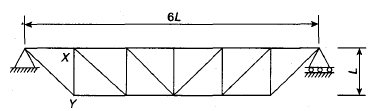Solution:
QUESTION: 11

What is the carry-over factor from A to B while using moment distribution for analyzing the given beam?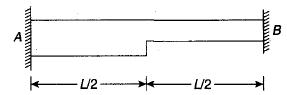Solution:

Carry-over factor means the amount by which moment applied on one support is developed on another support. For a fixed adjacent support in a uniform beam carry over factor is ½. But here, since the cross-section is larger on side A, thus, moment of Inertia will be higher leading to higher flexural rigidity. Hence, support A can bear larger moment in comparison to B.
Therefore, carry-over factor from A to B will be less than 0.5 while using moment distribution method.

QUESTION: 12

The influence line for force in member BC is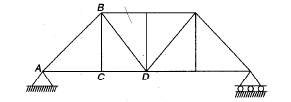Solution:

Member BC will act as a zero force member unless the unit load is between A and D. This is because member AC and CD are collinear horizontal members and any vertical force, developed in member BC cannot exist due to violation of Fy =0 equilibrium equation Moreover when the unit load is at C entire unit load will be balanced by member BC.
Therefore, the option D matches with the above description.

QUESTION: 13

What is the value of flexibility coefficient f12 for the continuous beam shown below?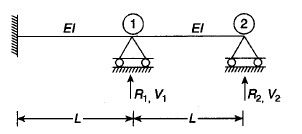Solution: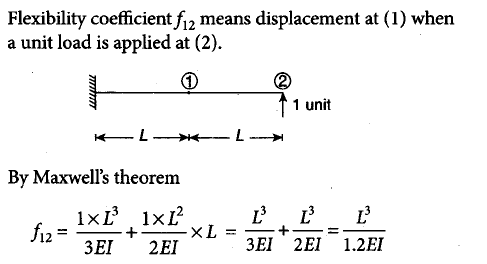QUESTION: 14

What is the ordinate of influence line at B for reaction RD in the figure below?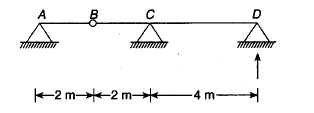Solution: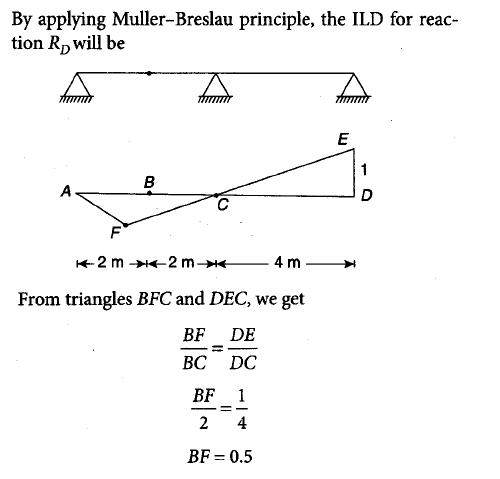QUESTION: 15

What is the value of vertical reaction at A for the frame shown below?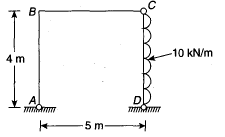Solution: